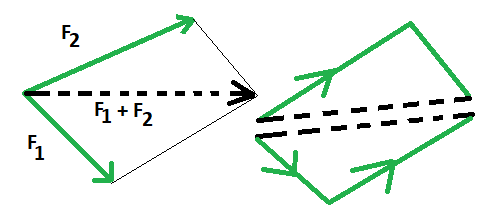# Average Force Formula

• Last Updated : 08 Mar, 2022

A force is commonly defined as a push or pull on an item due to contact of one item with another. When such an interaction occurs between two things, a force is exerted on both of the items. Such an interaction would lead to an exertion of force on each of the items. Multiple forces may be applied to a single object at times. In such a situation, average force is required to be calculated, which is discussed below.

### Average Force

The force generated by an item travelling at a given rate of speed, i.e. velocity, during a given time period is termed as an average force.The term ‘average’ is used to indicate that this velocity is not precisely measured or ‘instantaneous.’ As a result, the average force is equal to the product of mass and average velocity during the given duration. The amount and direction of average force are both vector variables.

Formula

If the time period is denoted by t, the force will be the periodicity of momentum change. When there are several time periods, the average force is the rate of variation of momentum.

It is expressed as the following formula:where,

• m depicts the mass of the object
• Vf and Vi are the final and initial values of velocity, respectively
• Δt is the time.

### Sample Problems

Question 1. A ball of mass 4 kg is rolled at 6 m/s for 12 seconds. Calculate its average force.

Solution:

Given: m = 4 kg, Vi = 0 m/s, Vf = 6 m/s, Δt = 12 s

Since, F =⇒ F = 4 (6 – 0)/12

F = 2 N

Question 2. A car of mass 600 kg travelling at 20 m/s comes to a complete halt in 5 seconds. What is the typical braking force?

Solution:

Given: m = 600 kg, Vi = 20 m/s, Vf = 0 m/s, Δt = 5 s

Since, v = u + at

⇒ a = v – u/t

⇒ a = 0 – 20/5 = -4 m/s

Since, F = ma

⇒ F = 600 x 4

F = 2400 N

Question 3. What would be the average force on an object of mass 50 kg, if it rolled at 6 m/s for 3 seconds.

Solution:

Given: m = 50 kg, Vi = 0 m/s, Vf = 6 m/s, Δt = 5 s

Since, F =⇒ F = 4 (6 – 0)/3

F = 8 N

Question 4. How much average force would one need to stop an object with momentum p in 0.5 seconds?

Solution:

Given: Initial momentum = Pi = p

Final momentum = Pf = 0

Average force = ΔP/Δt

= 0 – p/0.5

F = 2p N

Question 5. How much average force would one need to stop an object with momentum 4 in 2 seconds?

Solution:

Given: Initial momentum = Pi = 4

Final momentum = Pf = 2

Average force = ΔP/Δt

= 0 – 4/2

F = 2 N

My Personal Notes arrow_drop_up